## RD Sharma Class 9 Solutions Chapter 23 Graphical Representation of Statistical Data MCQS

These Solutions are part of RD Sharma Class 9 Solutions. Here we have given RD Sharma Class 9 Solutions Chapter 23 Graphical Representation of Statistical Data MCQS

Other Exercises

Question 1.
Which one of the following is not the graphical representation of statistical data:
(a) Bar graph
(b) Histogram
(c) Frequency polygon
(d) Cumulative frequency distribution
Solution:
Cumulative frequency distribution is not a graphical method.

Question 2.
In a frequency distribution, ogives are graphical representation of
(a) Frequency
(b) Relative frequency
(c) Cumulative
(d) Raw data
Solution:
Ogive is the graphical representation of cumulative frequency.

Question 3.
A frequency polygon is constructed by plotting frequency of the class interval and the
(a) upper limit of the class
(b) lower limit of the class
(c) mid value of the class
(d) any values of the class
Solution:
A frequency polygon is constructed by plotting frequency of the class interval and mid value of the class.

Question 4.
In a histogram the area of each rectangle is proportional to
(a) the class mark of the corresponding class interval
(b) the class size of the corresponding class interval
(c) frequency of the corresponding class interval
(d) cumulative frequency of the corresponding class interval
Solution:
In histogram, the area of each rectangle is proportional to frequency of the corresponding class intervals.

Question 5.
In the ‘less than’ type of ogive the cumulative frequency is plotted against
(a) the lower limit of the concerned class interval
(b) the upper limit of the concerned class interval
(c) the mid-value of the concerned class interval
(d) any value of the concerned class interval
Solution:
In the ‘less than’ type of ogive cumulative frequency is plotted against the upper limit of the concerned class interval,

Question 6.
In a histogram the class intervals or the groups are taken along
(a) Y-axis
(b) X-axis
(c) both of X-axis and Y-axis
(d) in between X and Y axis
Solution:
In histogram, the class intervals or the groups are taken along X-axisA

Question 7.
A histogram is a pictorial representation of the grouped data in which class intervals and frequency are respectively taken along
(a) vertical axis and horizontal axis
(b) vertical axis only
(c) horizontal axis only
(d) horizontal axis and vertical axis
Solution:
A histogram is a pictorial representation of the grouped data in which class interval and frequency are respectively taken along horizontal axis and vertical axis.

Question 8.
In a histogram, each class rectangle is constructed with base as
(a) frequency
(b) class-intervals
(c) range
(d) size of the class
Solution:
In a histogram each class rectangle is constructed with base as class intervals.

Hope given RD Sharma Class 9 Solutions Chapter 23 Graphical Representation of Statistical Data MCQS are helpful to complete your math homework.

If you have any doubts, please comment below. Learn Insta try to provide online math tutoring for you.

## RD Sharma Class 9 Solutions Chapter 23 Graphical Representation of Statistical Data Ex 23.3

These Solutions are part of RD Sharma Class 9 Solutions. Here we have given RD Sharma Class 9 Solutions Chapter 23 Graphical Representation of Statistical Data Ex 23.3

Other Exercises

Question 1.
Construct a histogram for the following data: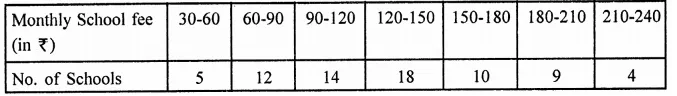Solution: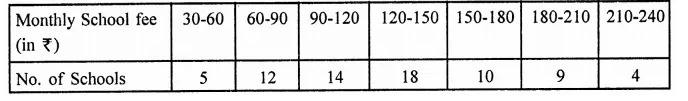Histogram is given below: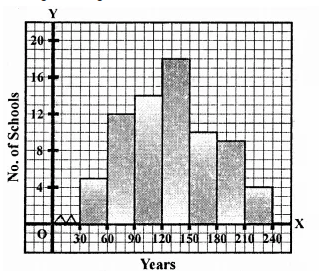Question 2.
The distribution of heights (in cm) of 96 children is given below. Construct a histogram and a frequency polygon on the same axes.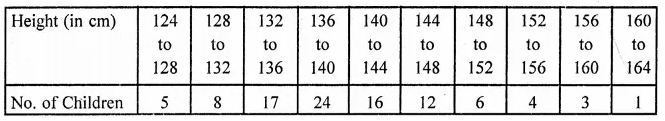Solution: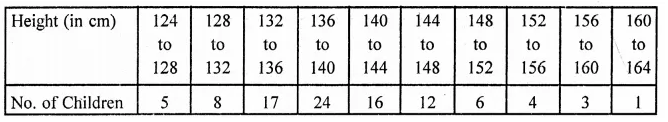Histogram and frequency polygon is given here.
Draw midpoints Of each rectangle at the top and join them to get a frequency polygon.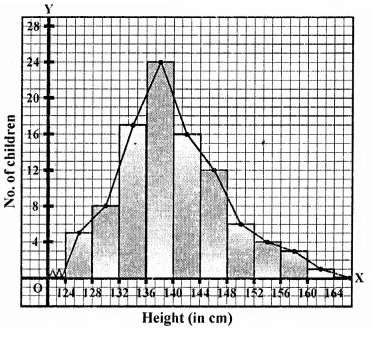Question 3.
The time taken, in seconds, to solve a problem by each of 25 pupils is as follows:
16, 20, 26, 27, 28, 30, 33, 37, 38, 40, 42, 43, 46, 46, 46, 48, 49, 50, 53, 58, 59, 60, 64, 52, 20
(a) Construct a frequency distribution for these data, using a class interval of 10 seconds.
(b) Draw a histogram to represent the frequency distribution.
Solution:
Lowest observation = 16,
Highest observation = 64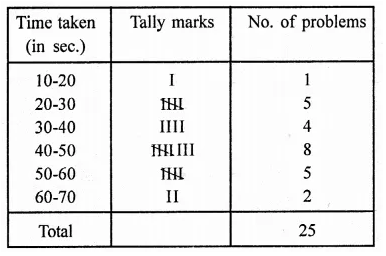Below is given the histogram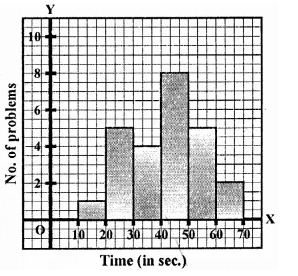Question 4.
Draw, in the same diagram, a histogram and a frequency polygon to represent the following data which shows the monthly cost of living index of a city in a period of 2 years: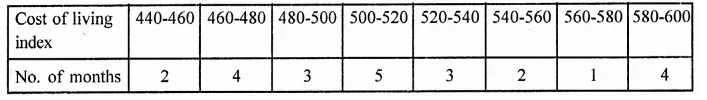Solution: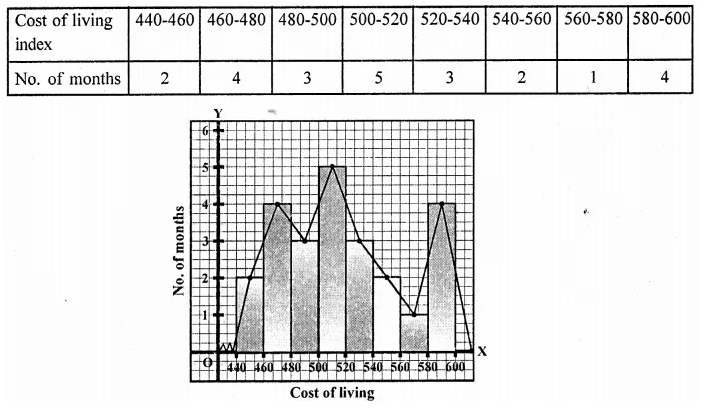Question 5.
The following is the distribution of total household expenditure (in Rs.) of manual worker in a city: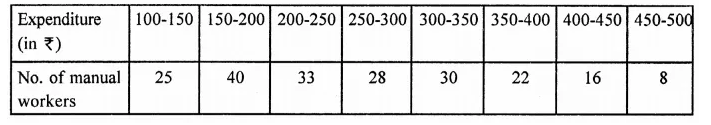Draw a histogram and a frequency polygon representing the above data.
Solution: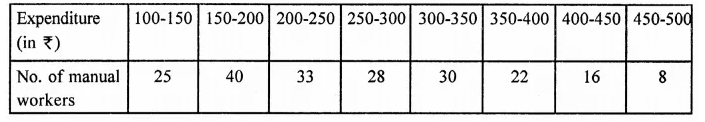Here is given histogram and frequency polygon.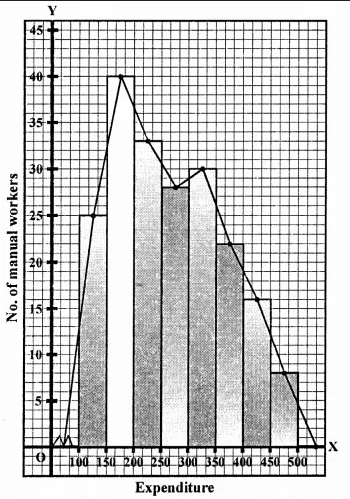Question 6.
The following table gives the distribution of IQ’s (intelligence quotients) of 60 pupils of class V in a school: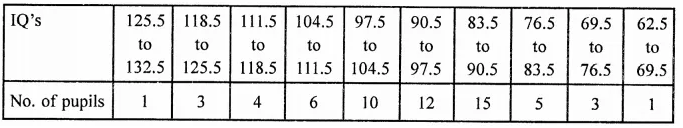Draw a frequency polygon for the above data.
Solution: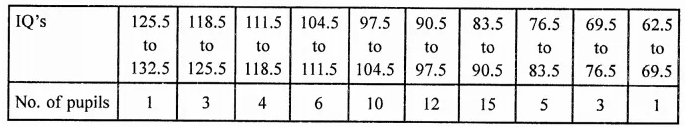Now plot the (mid point, frequency) on the graph and join them to get a frequency polygon.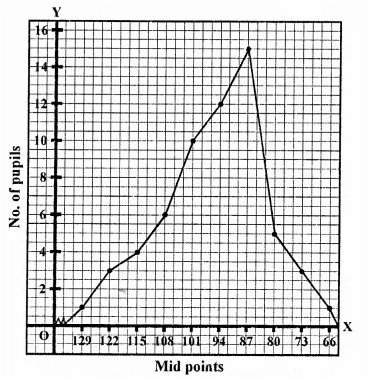Question 7.
Draw a histogram for the daily earnings of 30 drug stores in the following table: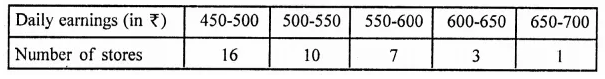Solution: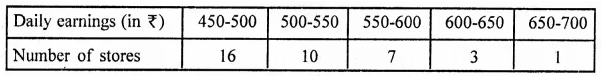Hisotgram is given below: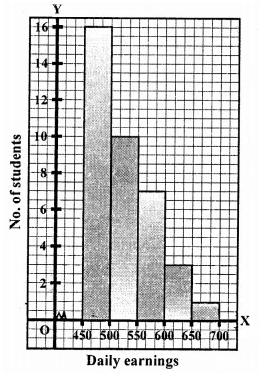Question 8.
The monthly profits (in t) of 100 shops are distributed as follows: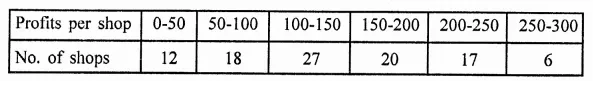Draw a histogram for the data and show the frequency polygon for it.
Solution: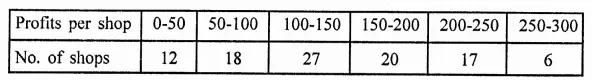Histogram and frequency polygon is given below: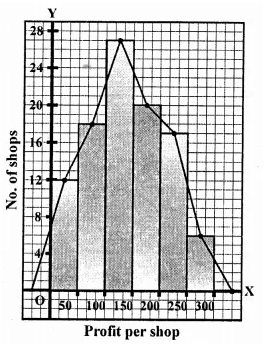Hope given RD Sharma Class 9 Solutions Chapter 23 Graphical Representation of Statistical Data Ex 23.3 are helpful to complete your math homework.

If you have any doubts, please comment below. Learn Insta try to provide online math tutoring for you.

## RD Sharma Class 9 Solutions Chapter 23 Graphical Representation of Statistical Data Ex 23.2

These Solutions are part of RD Sharma Class 9 Solutions. Here we have given RD Sharma Class 9 Solutions Chapter 23 Graphical Representation of Statistical Data Ex 23.2

Other Exercises

Question 1.
Explain the reading and interpretation of bar graphs.
Solution:
Reading and interpretation of the bar graph:
The first step in reading a bar graph is to know what it represents or what is the information given by it. For this, we read the captions which are generally written just below the horizontal line (x-axis) and adjacent to vertical line (y-axis). After knowing that what does a bar graph represent we read the scale so that we can know the precise values in the given data.
After reading a bar graph one must be able to draw certain conclusions from it. Drawing some conclusions from a given bar graph means interpretation of the bar graph.

Question 2.
(i) What information is given by the bar graph?
(ii) In which year the export is minimum?
(iii) In which year the import is maximum?
(iv) In which year the difference of the values of export and import is maximum?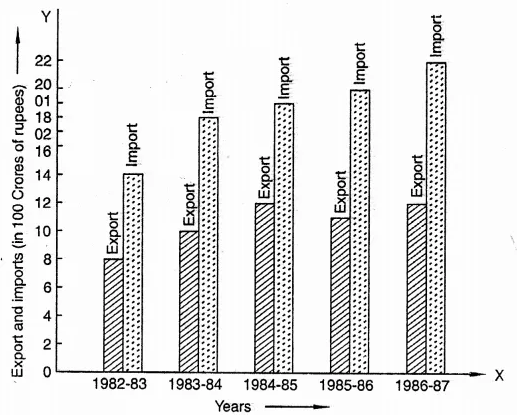Solution:
After reading the bar graph. We find:
(i) Export and import in 100 crores of rupees year wise.
(ii) In the year 1982-83, export is minimum i.e. 100 crores.
(iii) In the year 1986-87, import is maximum i.e. 22 hundred crores.
(iv) In the year 1986-87, the difference of the values of import and export is maximum i.e. 22 – 12 = 10 x 100 crore.

Question 3.
The following bar graph show’s the results of an annual examination in a secondary school.
Read the bar graph and choose the correct alternative in each of the following:
(i) The pair of classes in which the results of boys and girls are inversely proportional are:
(a) VI, VIII (b) VI, IX (c) VIII, IX (d) VIII, X
(ii) The class having the lowest failure rate of girls is
(a) VII (b) X (c) IX (d) VIII
(iii) The class having the lowest pass rate of students is
(a) VI (b) VII (c) VIII (d) IX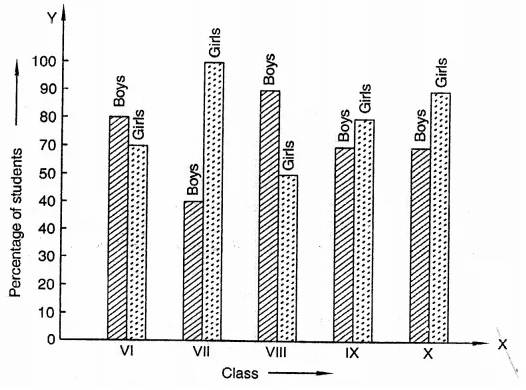Solution:
After reading the given bar graph, we find that
(i) The pair of classes in which the results of boys and girls are inversely proportional is VI and IX as in VI, 80% boys and 70% girls and in IX, 70% boys and 80% girls are the results.
(ii) Lowest failure of girls of students is VII as pass % is 100%.
(iii) Class having the lowest pass rate is VII boys as it is 50% only.

Question 4.
The following data gives the number (in thousands) of applicants registered with an Employment Exchange during, 1995-2000: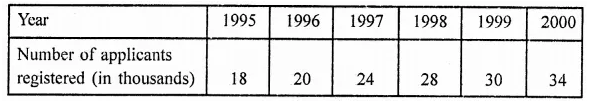Construct a bar graph to represent the above data.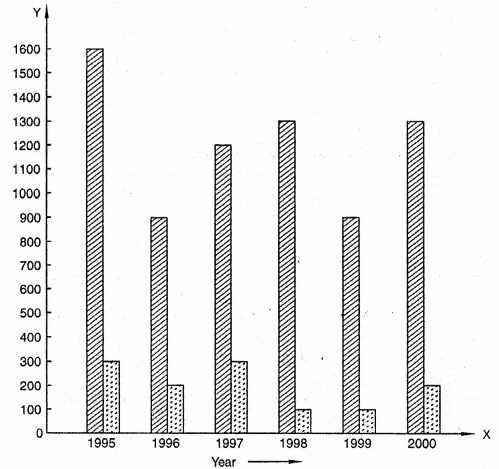Solution: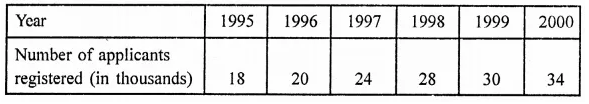Bar graph of the above is given below: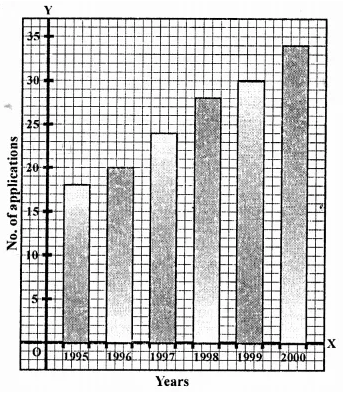Question 5.
The production of saleable steel in some of the steel plants of our country during 1999 is given below: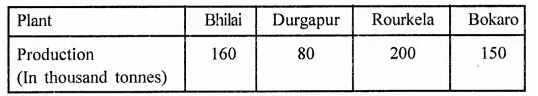Construct a bar graph to represent the above data on a graph paper by using the scale 1 big divisions = 20 thousands tonnes.
Solution: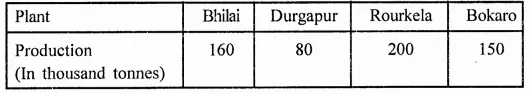Bar graph of the above data is given below: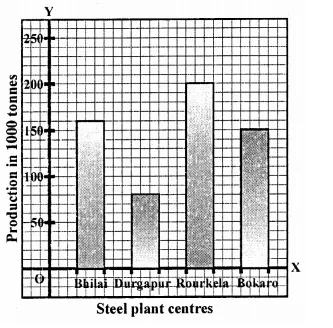Question 6.
The following table gives the route length (in thousand kilometres) of the Indian Railways in some of the years: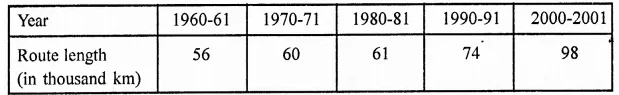Represent the above data with the help of a bar graph.
Solution: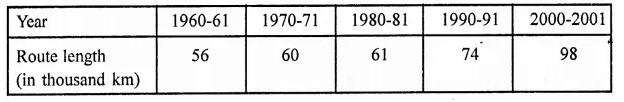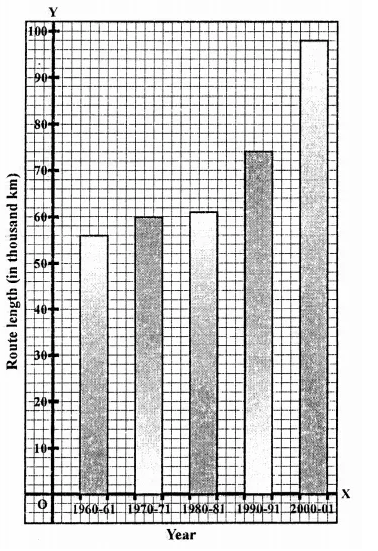Question 7.
The following data gives the amount of loans (in crores of rupees) disbursed by a bank during some years: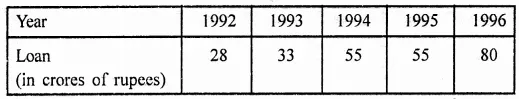(i) Represent the above data with the help of a bar graph.
(ii) With the help of the bar graph, indicate the year in which amount of loan is not increased over that of the preceding year.
Solution: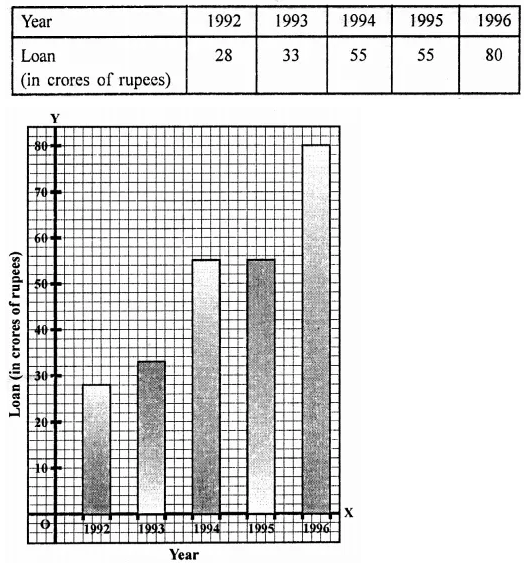Question 8.
The following table shows the interest paid by a company (in lakhs):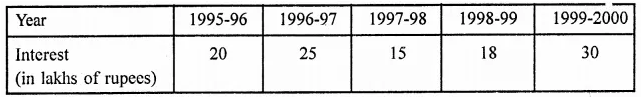Draw the bar graph to represent the above information.
Solution: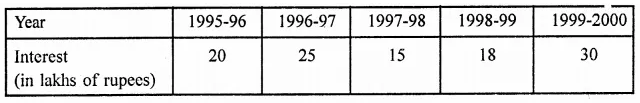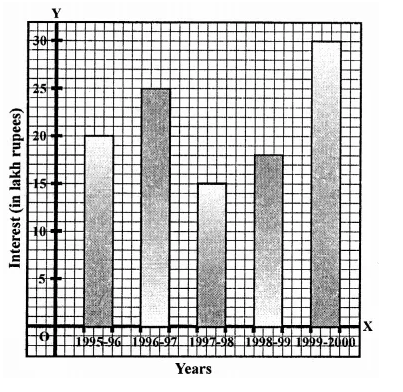Question 9.
The following data shows the average age of men in various countries in a certain year: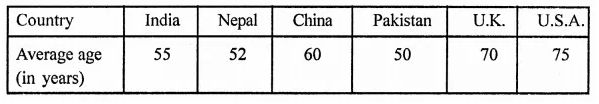Represent the above information by a bar graph.
Solution: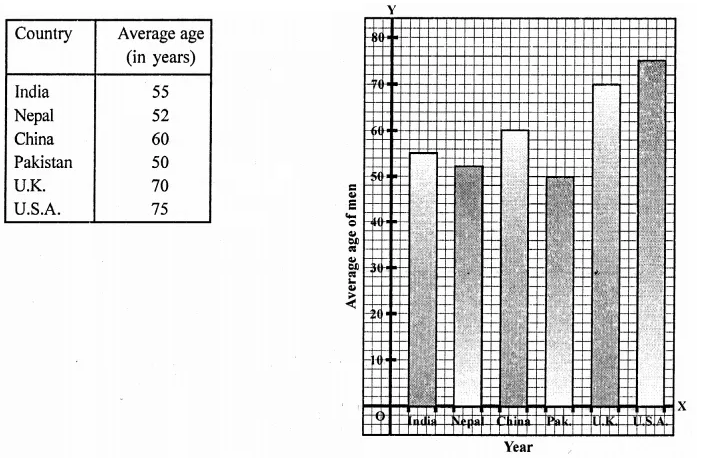Question 10.
The following data gives the production of foodgrains (in thousand tonnes) for some years: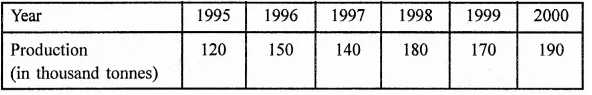Represent the above data with the help of a bar graph.
Solution: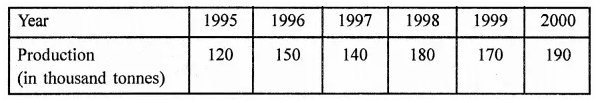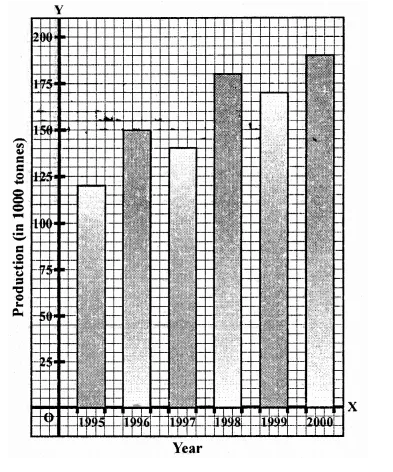Question 11.
The following data gives the amount of manure (in thousand tonnes) manufactured by a company during some years: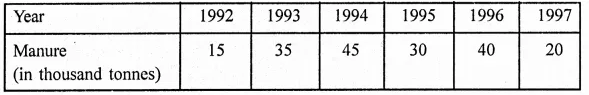(i) Represent the above data with the help of a bar graph.
(ii) Indicate with the help of the bar graph the year in which the amount of manufactured by the company was maximum.
(in) Choose the correct alternative:
The consecutive years during which there was maximum decrease in manure production are:
(a) 1994 and 1995
(b) 1992 and 1993
(c) 1996 and 1997
(d) 1995 and 1996
Solution: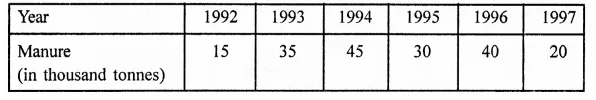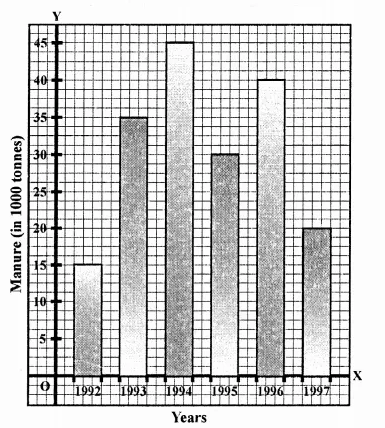(ii) The maximum amount of manure manufactured in the year 1994, i.e. 45 thousand tonnes.
(iii) There was maximum decrease in manure production in 1996 and 1997.

Question 12.
The following data gives the demand estimates of the Government of India, Department of Electronics for the personnel in the Computer sector during the Eighth Plan period (1990-95):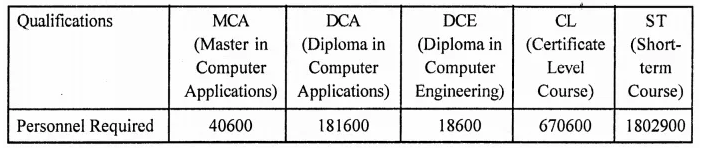Represent the data with the help of a bar graph. Indicate with the help of the bar graph the course where estimated requirement is least.
Solution: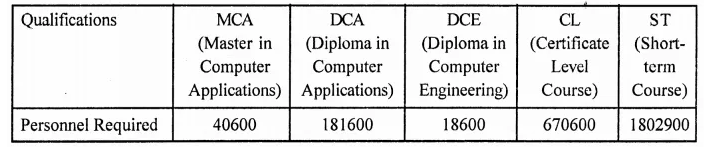The course where requirement is least is DCE.

Question 13.
The income and expenditure for 5 years of a family is given in the following data: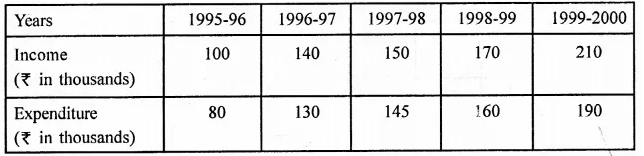Represent the above data by a bar graph.
Solution: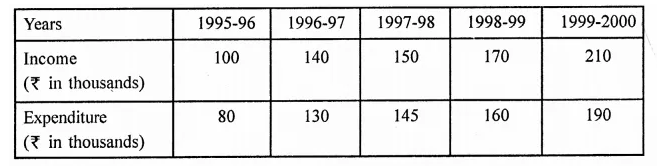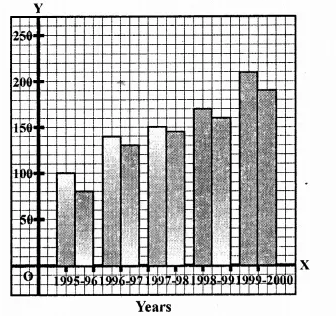Question 14.
The investment (in ten crores of rupees) of Life Insurance Corporation of India in different sectors are given below: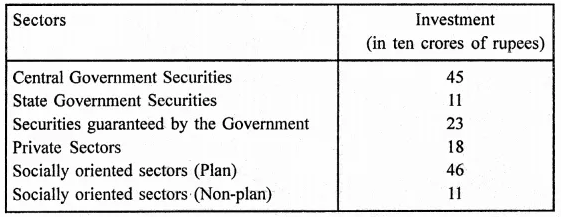Represent the above data with the help of a bar graph.
Solution: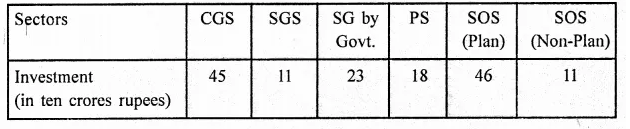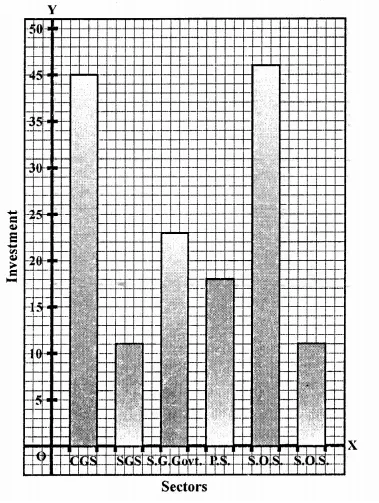Question 15.
The following data gives the value (in crores of rupees) of the Indian export of cotton textiles for different years: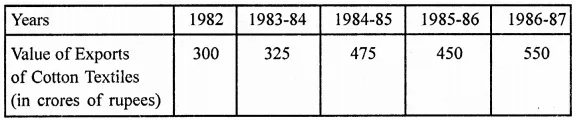Represent the above data with the help of a bar graph. Indicate with the help of a bar graph the year in which the rate of increase in exports is maximum over the preceding year.
Solution: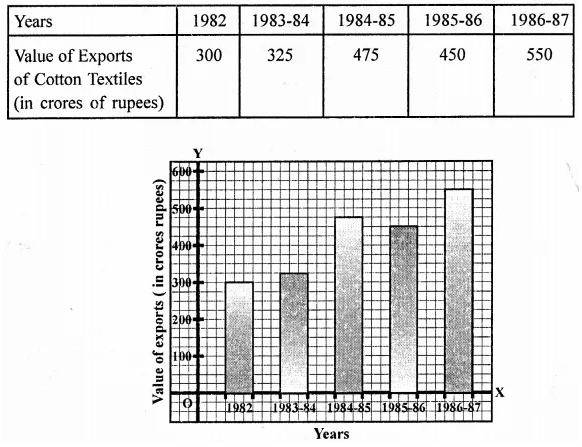Question 16.
The following table gives the quantity of good (in crore tonnes)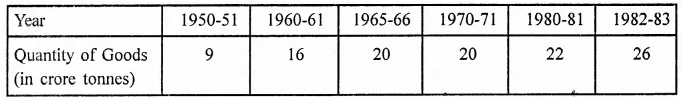Represent this information with the help of a bar graph.
Explain through the bar graph if the quantity of goods carried by the Indian Railways in 1965-66 is more than double the quantity of goods carried in the year 1950-51.
Solution: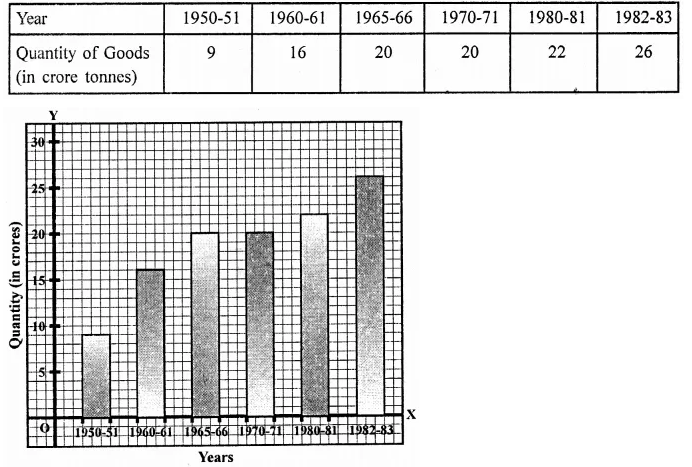Question 17.
The production of oil (in lakh tonnes) in some of the refineries in India during 1982 was given below: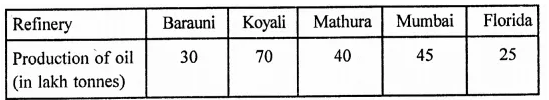Construct a bar graph to represent the above data so that the bars are drawn horizontally.
Solution: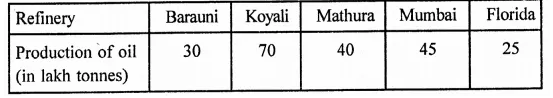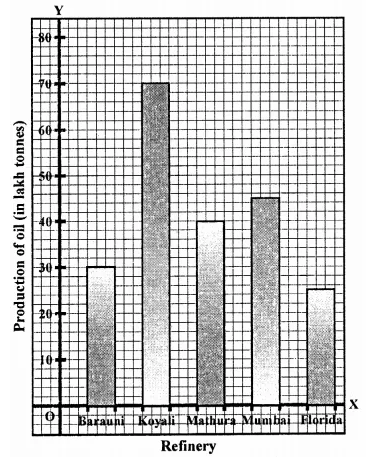Question 18.
The expenditure (in 10 crores of rupees) on health by the Government of India during the various five year plans is shown below: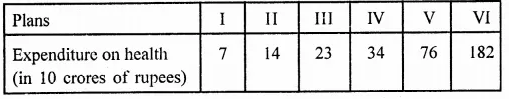Construct a bar graph to represent the above data.
Solution: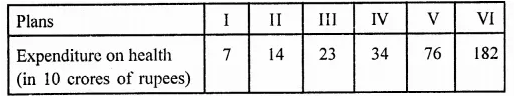Hope given RD Sharma Class 9 Solutions Chapter 23 Graphical Representation of Statistical Data Ex 23.2 are helpful to complete your math homework.

If you have any doubts, please comment below. Learn Insta try to provide online math tutoring for you.

## RD Sharma Class 9 Solutions Chapter 23 Graphical Representation of Statistical Data Ex 23.1

These Solutions are part of RD Sharma Class 9 Solutions. Here we have given RD Sharma Class 9 Solutions Chapter 23 Graphical Representation of Statistical Data Ex 23.1

Other Exercises

Question 1.
The following table shows the daily production of T.V. sets in an industry for 7 days of a week: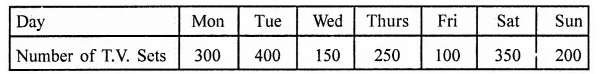Represent the above information by a pictograph.
Solution: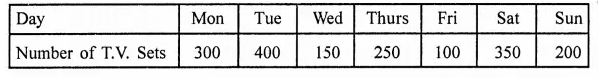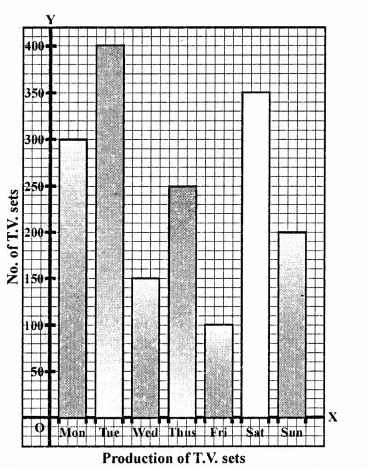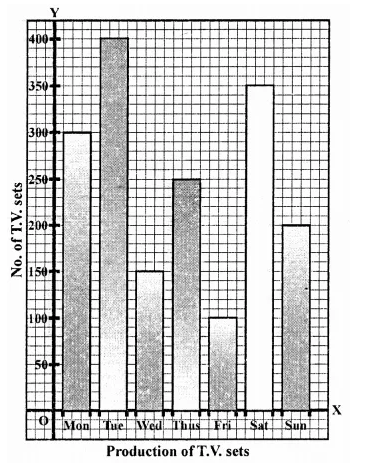Question 2.
The following table shows the number of Maruti cars sold by five dealers in a particular month: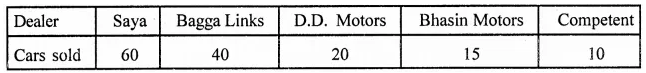Represent the above information by a pictograph.
Solution: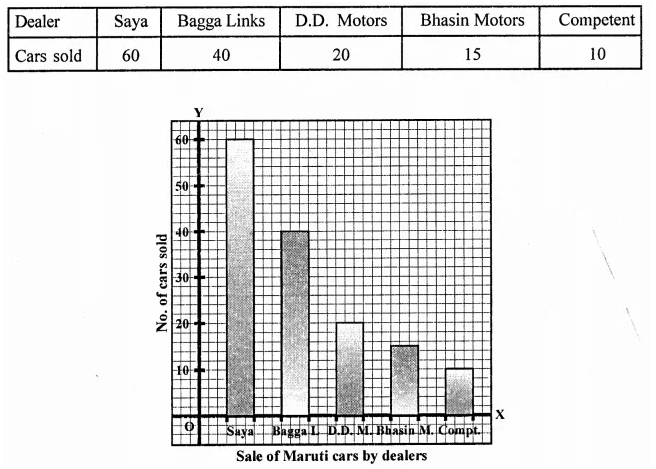Question 3.
The population of Delhi State in different census years is as given below: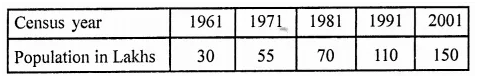Represent the above information with the help of a bar graph.
Solution: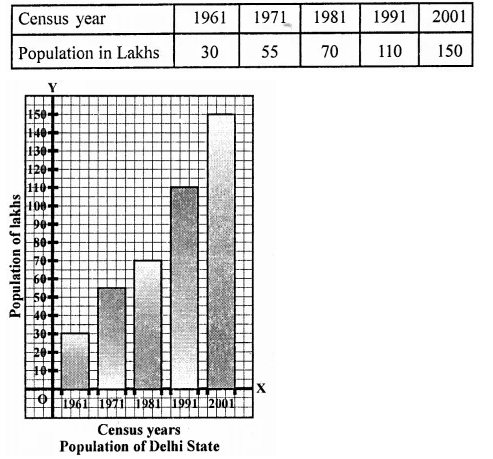Question 4.
Read the bar graph shown in Figure and answer the following questions: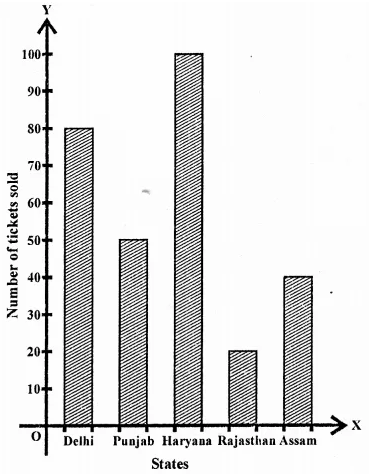(i) What is the information given by the bar graph?
(ii) How many tickets of Assam State Lottery were sold by the agent?
(iii) Of which state, were the maximum number of tickets sold?
(iv) State whether true or false.
The maximum number of tickets sold is three times the minimum number of tickets sold.
(v) Of which state were the minimum number of tickets sold?
Solution:
(i) Sale of lottery tickets if different states.
(ii) 40 tickets of Assam state lottery were sold.
(iii) Maximum number of lottery tickets were sold of Haryana state.
(iv) Maximum tickets were 100 and minimum = 20 which are not three time. False.
(v) Minimum Lottery tickets of 10 Rajasthan will sold.

Question 5.
Study the bar graph representing the number of persons in various age groups in a town shown in figure. Observe the bar graph and answer the following questions:
(i) What is the percentage of the youngest age-group persons over those in the oldest age group?
(ii) What is the total population of the town?
(iii) What is the number of persons in the age-group 60-65?
(iv) How many persons are more in the age-group 10-15 than in the age group 30-35?
(v) What is the age-group of exactly 1200 persons living in the town?
(vi) What is the total number of persons living in the town in the age-group 50-55?
(vii) What is the total number of persons living in the town in the age-groups 10-15 and 60-65?
(viii) Whether the population in general increases, decreases or remains constant with the increase in the age-group.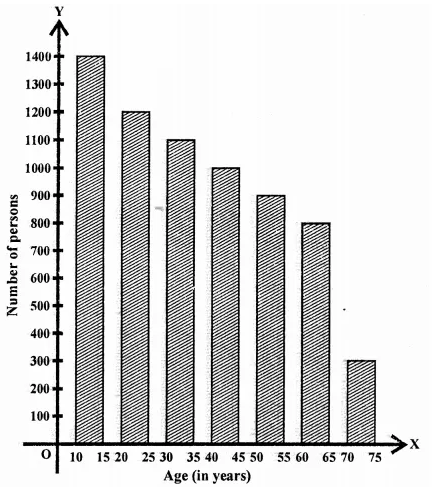Solution:
(i) Youngest age group = 300 and oldest age group = 1400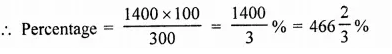(ii) Total population = 1400 + 1200 + 1100 + 1000 + 900 + 800 + 300 – 6700
(iii) In age group of 60-65, number of persons = 800
(iv) Difference of person in the age group 10-15 and of 30-35 = 1400 – 1100 = 300
(v) 1200 persons are in the age group 20-25
(vi) In age group 50-55 number of persons are 900
(vii) Total number of person of the age group 10-15 and
60-65 = 1400 + 800 = 2200
(viii)Population is decreasing with the age-group.

Question 6.
Read the bar graph shown in figure and answer the following questions:
(i) What is the information given by the bar graph?
(ii) What was the number of commercial banks in 1977?
(iii) What is the ratio of the number of commercial banks in 1969 to that in 1980?
(iv) State whether true or false:
The number of commercial banks in 1983 is less than double the number of commercial banks in 1969.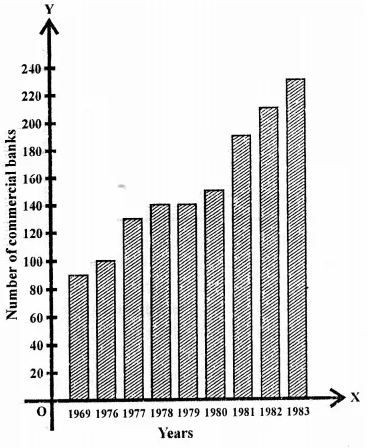Solution:
(i) The information is number of commercial banks in India during same years.
(ii) In 1977, commercial banks were 130.
(iii) Ratio of banks in 1969 to 1980 = 90 : 150 = 3:5
(iv) Number of banks in 1983 = 230 and number of banks in 1969 = 90
∴ Number of banks in 1983 is less than double the number of banks in 1969 – False.

Question 7.
Given below is the bar graph indicating the marks obtained out of 50 in mathematics paper by 100 students. Read the bar graph and answer the following questions: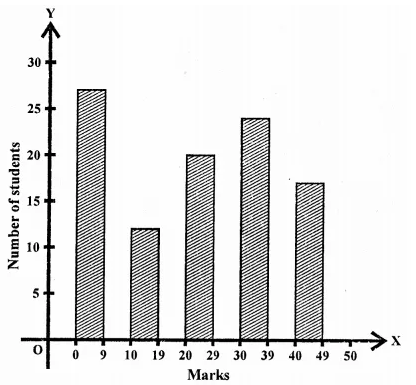(i) It is decided to distribute work books on mathematics to the students obtaining less than 20 marks, giving one workbook to each of such students. If a work book costs T5, what sum is required to buy the work books?
(ii) Every student belonging to the highest mark group is entitled to get a prize of ? 10. How much amount of money is required for distributing the prize money?
(iii) Every student belonging to the lowest mark-group has to solve 5 problems per day. How many problems, in all, will be solved by the students of this group per day?
(iv) State whether true or false. .
(a) 17% students have obtained marks ranging from 40 to 49.
(b) 59 students have obtained marks ranging from 10 to 29.
(v) What is the number of students getting less than 20 marks?
(vi) What is the number of students getting more than 29 marks?
(vii) What is the number of students getting marks between 9 and 40?
(viii)What is the number of students belonging to the highest mark group?
(ix) What is the number of students obtaining more than 19 marks?
Solution:
(i) Students getting less than 20 marks = 27 + 12 = 39
Cost of 1 work book = ₹5
∴ Total cost = 39 x 5 = ₹195
(ii) No. of students getting highest mark group = 17
∴ Amount paid at the rate of Rs. 10 to each = 10 x 17 = Rs. 170
(iii) Lowest mark group = 27
∴ Total number of problems given = 27 x 5 = 135
(iv) (a) True (b) False
(v) No. of students getting less then 20 marks = 27 + 12 = 39
(vi) Students getting more than 29 marks = 24 + 17 = 41
(vii) Students getting marks between 9 and 40 = 12 + 20 + 24 = 56
(viii) Students to the highest mark group = 17
(ix) Students setting more than 19 marks = 20 + 24 + 17 = 61

Question 8.
(i) What is the information given by the bar graph?
(ii) State each of the following whether true or false.
(a) The number of government companies in 1957 is that of 1982 is 1 : 9.
(b) The number of government companies have decreased over the year 1957 to 1983.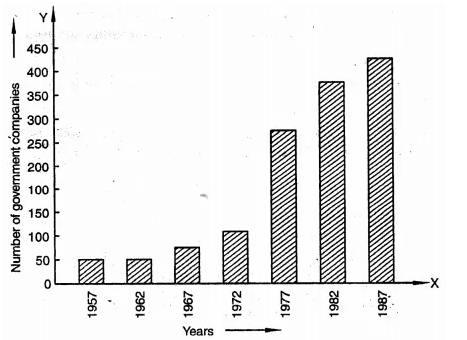Solution:
(i) Number of government companies in India during some years.
(ii) (a) False (b) False
(a) 50 : 400 = 1:8 and (b) companies are increasing not decreasing.

Question 9.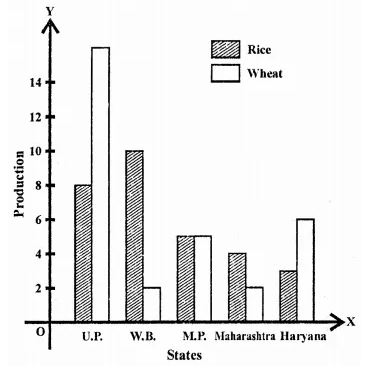(i) What information is given by the bar graph?
(ii) Which state is the largest producer of rice?
(iii) Which state is the largest producer of wheat?
(iv) Which state has total production of rice and wheat as its maximum?
(v) Which state has the total production of wheat and rice minimum?
Solution:
(i) Production of rice and wheat in different states of India.
(ii) West Bengal is the largest state of production of rice.
(iii) Largest producer of wheat is U.P.
(iv) Maximum production of wheat and rice is U.P.
(v) Minimum production of wheat and rice is Maharashtra.

Question 10.
The following bar graph represents the heights (in cm) of 50 students of Class XI of a particular school. Study the graph and answer the following questions: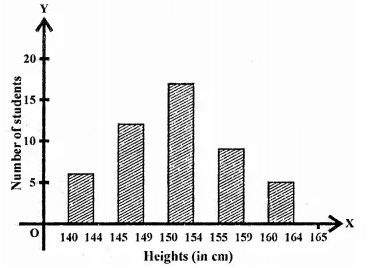(i) What percentage of the total number of students have their heights more than 149 cm?
(ii) How many students in the class are in the range of maximum height of the class?
(iii) The school wants to provide a particular type of tonic to each student below the height of 150 cm to improve his height. If the cost of the tonic for each student comes out to be Rs. 55, how much amount of money is required?
(iv) How many students are in the range of shortest height of the class?
(v) State whether true or false:
(a) There are 9 students in the class whose heights are in the range of 155-159 cm.
(b) Maximum height (in cm) of a student in the class is 17.
(c) There are 29 students in the class whose heights are in the range of 145-154 cm.
(d) Minimum height (in cm) of a student is the class is in the range of 140-144 cms.
(e) The number of students in the class having their heights less than 150 cm is 12.
(f) There are 14 students each of whom has height more than 154 cm.
Solution:
(i) No. of students having more than height of 149 cm = 17 + 9 + 5 = 31
Percentage = $$\frac { 31 }{ 50 }$$ x 100 = 62%
(ii) No. of students in the range of maximum height = 5
(iii) No. of students below the height of 150 cm = 7 + 12 = 19
Cost of tonic for each student = ₹55
∴ Total cost = ₹55 x 19 = ₹1045
(iv) No. of students in the shortest height = 7
(v) (a) True
(b) False
(c) True
(d) True
(e) False
(f) True

Question 11.
(i) What information is given by the bar graph?
(ii) What was the production of cement in the year 1980-81?
(iii) What is the minimum and maximum productions of cement and corresponding years?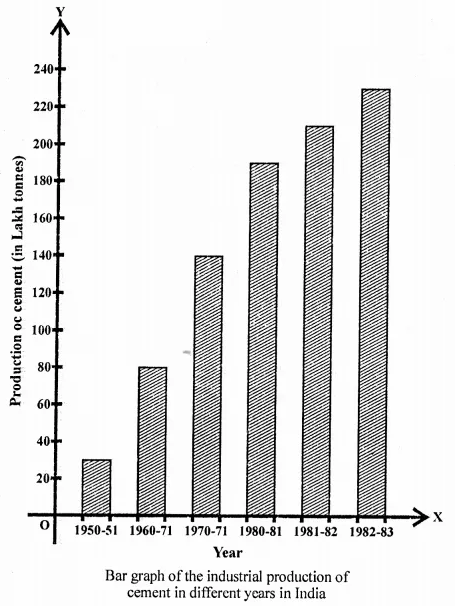Solution:
(i) Industrial production of cement in different years in India.
(ii) In the year 1980-81, the production was 186 lakh tonnes.
(iii) Minimum production was 30 lakh tonnes in 1950-51 and maximum production was 232 lakh tonnes in 1982-83.

Question 12.
The bar graph shown in the figure represents the circulation of newspapers in 10 languages. Study the bar graph and answer the following questions: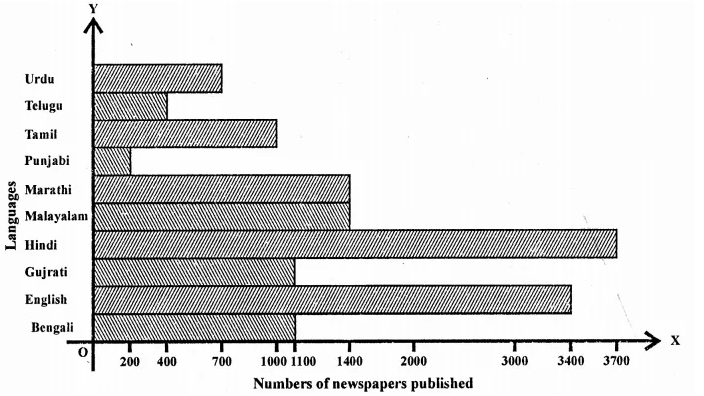(i) What is the total number of newspapers published in Hindi, English, Urdu, Punjabi and Bengali?
(ii) What percent is the number of news papers published in Hindi of the total number of newspapers?
(iii) Find the excess of the number of newspapers published in English over those published in Urdu,
(iv) Name two pairs of languages which publish the same number of newspapers.
(v) State the languages in which the smallest number of newspapers are published.
(vi) State the language in which the largest number of newspapers are published.
(vii) State the language in whih the number of newspapers published is between 2500 and 3500.
(viii)State whether true or false:
(a) The number of newspapers published in Malayalam and Marathi together is- less than those published in English.
(b) The number of newspapers published in Telugu is more than those published in Tamil.
Solution:
(i) Total number of newspapers in Hindi, English, Urdu, Punjabi, and Bengali
= 3700 + 3400 + 700 + 200 + 1100 = 9100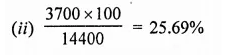(iii) Difference in English and Urdu = 3400 – 700 = 2700
(iv) Malayalam and Marathi, Bengali and Gujrati
(v) Punjabi (200)
(vi) Hindi (3700)
(vii) English (3400)
(viii)(a) True (b) False

Question 13.
Read the bar graph given in figure and answer the following questions:
(i) What information is given by the bar graph?
(ii) What was the crop-production if rice in 1970-71?
(iii) What is the difference between the maximum and minimum production of rice?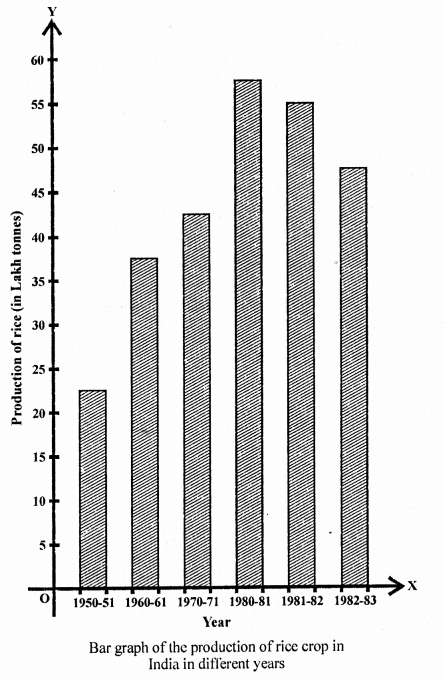Solution:
(i) Production of rice in India in different years.
(ii) Crop production in 1970-71 was 42.5 lakh tonnes.
(iii) Differences in maximum and minimum production of rice = 55 – 22 lakh tonnes = 33 lakh tonnes

Question 14.
Read the bar graph given in figure and answer the following questions: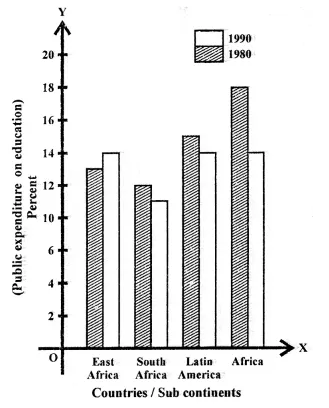(i) What information does it give?
(ii) in which part the expenditure on education is maximum in 1980?
(iii) In which part the expenditure has gone up from 1980 to 1990?
(iv) In which part the gap between 1980 and 1990 is maximum?
Solution:
(i) Public expenditure on education by vertices sub-continents in 1980 and 1990.
(ii) Maximum expenditure on education in 1980 was in Africa.
(iii) The expenditure from 1980 to 1990 was gone up in East Africa.
(iv) Maximum gap between 1980 and 1990 was in Africa.

Question 15.
Read the bar graph given in the figure and answer the following questions: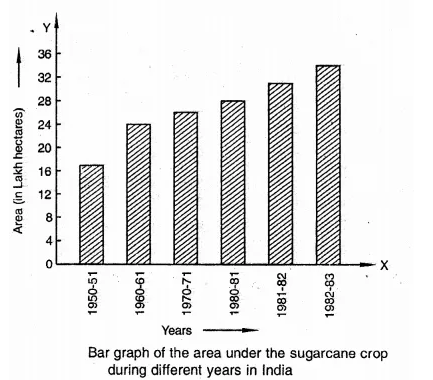(i) What information is given by the bar graph?
(ii) In whicli years the areas under the sugarcane crop were the maximum and the minimum?
(iii) State whether true or false:
The area under the sugarcane crop in the year 1982-83 is three times that of the year 1950-51.
Solution:
(i) Areas under sugarcane crop during different years in India.
(ii) Maximum sugarcane crop was in 1982-83 and minimum in 1950-51.
(iii) False.

Question 16.
Read the bar graph given in the figure and answer the following questions: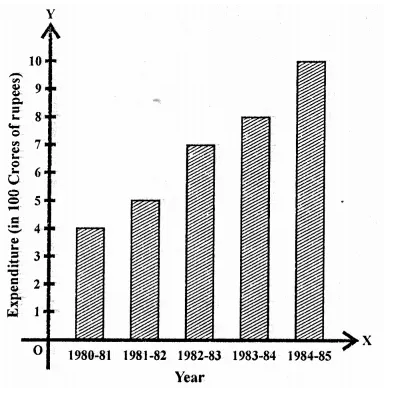(i) What information is given by the bar graph?
(ii) What was the expenditure on health and family planning in the year 1982-83?
(iii) In which year is the increase in expenditure maximum over the expenditure in previous year? What is the maximum increase?
Solution:
(i) Expenditure on health and family planning sixth five year plan in India.
(ii) Expenditure during 1982-83 was 700 crore of rupees.
(iii) Maximum increase in 1984-85 was them in previous year and difference 10.2 – 8 = 220 crores.

Question 17.
Read the bar graph given in the figure and answer the following questions:
(i) What is the information given by the bar graph?
(ii) What is the number of families having 6 members?
(iii) How many members per family are there in the maximum number of families? Also tell the number of such families.
(iv) What are the number of members per family for which the number of families are equal? Also, tell the number of such families?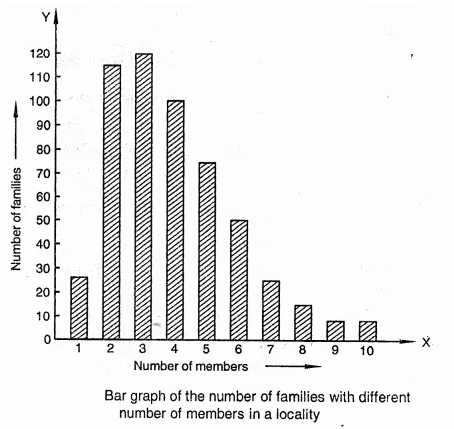Solution:
(i) Number of families with different number of members in a locality.
(ii) Number of families having 6 members are 50.
(iii) Maximum number of families are of 3 members family and there are 120.
(iv) Number of families having 9 members and having 10 members are equal and each is 5.

Question 18.
Read the bar graph given in the figure and answer the following questions: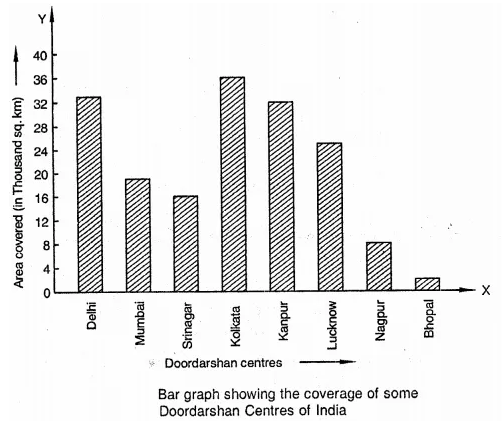(i) What information is given by the bar graph?
(ii) Which Doordarshan centre covers maximum area? Also tell the covered area.
(iii) What is the difference between the areas covered by the centres at Delhi and Bombay?
(iv) Which Doordarshan centres are in U.P. State? What are the areas covered by them?
Solution:
(i) Coverage of some Doordarshan centres of India.
(ii) Doordarshan kender Kolkata covers maximum area which is 36000 sq. km.
(iii) Difference of areas covered by Delhi and Bombay centres = 32000 – 18000 = 14000 sq. km.
(iv) In U.R Doordarshan centres are Kanpur (32000 sq. km) and Lucknow (25000 sq. km).

Hope given RD Sharma Class 9 Solutions Chapter 23 Graphical Representation of Statistical Data Ex 23.1 are helpful to complete your math homework.

If you have any doubts, please comment below. Learn Insta try to provide online math tutoring for you.

## RD Sharma Class 9 Solutions Chapter 22 Tabular Representation of Statistical Data MCQS

These Solutions are part of RD Sharma Class 9 Solutions. Here we have given RD Sharma Class 9 Solutions Chapter 22 Tabular Representation of Statistical Data MCQS

Other Exercises

Mark the correct alternative in each of the following:
Question 1.
Tally marks are used to find
(a) class intervals
(b) range
(c) frequency
(d) upper limits
Solution:
Frequency (c)

Question 2.
The difference between the highest and lowest values of the observations is called
(a) frequency
(b) mean
(c) range
(d) class intervals
Solution:
range (c)

Question 3.
The difference between the upper limit and the lower class limits is called
(a) mid-points
(b) class size
(c) frequency
(d) mean
Solution:
class size (b)

Question 4.
In the class intervals 10-20, 20-30, 20 is taken in
(a) the interval 10-20
(b) the interval 20-30
(c) both interval 10-20, 20-30
(d) none of the intervals
Solution:
the interval 20-30 (b)

Question 5.
In a frequency distribution, the mid value of a class is 15 and the class intervals is 4. The lower limit of the class is
(a) 10
(b) 12
(c) 13
(d) 14
Solution:
Mid value = 15 and class interval is 4
∴ Lower limit = 15 – $$\frac { 4 }{ 5 }$$ = 15 – 2 = 13 (c)

Question 6.
The mid-value of a class interval is 42. If the class size is 10, then the upper and lower limits of the class are
(a) 47 and 37
(b) 37 and 47
(c) 37.5 and 47.5
(d) 47.5 and 37.5
Solution:
Mid value = 42 and class size =10
∴ Upper limit = 42 + $$\frac { 10 }{ 2 }$$ = 42 + 5 = 47
and lower limit = 42 – $$\frac { 10 }{ 2 }$$ = 42 – 5 = 37
Upper class limit and lower class limits are 47, 37 (a)

Question 7.
The number of times a particular item occurs in a given data is called its
(a) variation
(b) frequency
(c) cumulative frequency
(d) class-size
Solution:
frequency (b)

Question 8.
The width of each of nine classes in a frequency distribution is 2.5 and the lower class boundary of the lowest class 10.6. Then the upper class boundary of the highest class is
(a) 35.6
(b) 33.1
(c) 30.6
(d) 28.1
Solution:
Width of each class = 2.5
No. of classes = 9
Lower class boundary of the lowest class = 10.6
∴ Upper class limit of highest class = 10.6 + 9 x 2.5
= 10.6 + 22.5 = 33.1 (b)

Question 9.
The following marks were obtained by the students in a test:
81, 72, 90, 90, 86, 85, 92, 70, 71, 83, 89, 95, 85, 79, 62
The range of the marks is
(a) 9
(b) 17
(c) 27
(d) 33
Solution:
Marks are 81, 72, 90, 90, 86, 85, 92, 70, 71, 83, 89, 95, 85, 79, 62
Here highest marks = 95
and lowest marks = 62
∴ Range = highest marks – lowest marks = 95 – 62 = 33 (d)

Question 10.
Tallys are usually marked in a bunch of
(a) 3
(b) 4
(c) 5
(d) 6
Solution:
4 i.e. IIII (b)

Question 11.
Let l be the lower class limit of a class- interval in a frequency distribution and m be the mid-point of the class. Then, the upper class limit of the class is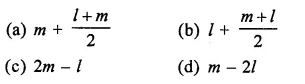Solution:
l is the lower class limit of a class interval
m is the mid point of the class
m = $$\frac { u+l }{ 2 }$$
⇒ 2m = u+ l ⇒ u = 2m – l
Then, upper class limit = 2 m – l (c)

Hope given RD Sharma Class 9 Solutions Chapter 22 Tabular Representation of Statistical Data MCQS are helpful to complete your math homework.

If you have any doubts, please comment below. Learn Insta try to provide online math tutoring for you.